# Hazen-Williams vs Darcy-Weisbach

#### Engineering Piping

Both Hazen-Williams and Darcy-Weisbach equations compute head loss due to friction in a pipe. Hazen-Williams coefficient is a function of only the pipe roughness vs Darcy-Weisbach which considers internal roughness, velocity, and hydraulic slope. In this article, you will learn more about the Hazen-Williams and Darcy-Weisbach equations, and compare the use of both with each other as well as other formulas.

## Hazen-Williams Equation

The Hazen-Williams equation stems from using empirical methods in predicting head loss in a water pipe when flow is turbulent. Before the advent of more sophisticated models such as Darcy-Weisbach, the use of Hazen-Williams was predominant amongst engineers. Moreover, it predicts frictional head loss (hf) as a function of the pipe length (L), friction coefficient (C), flow rate (gpm), and pipe internal diameter (d).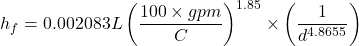The equation above is the expression for the head loss in imperial units. Alternatively, in S.I. units, the symbol for flow rate (Q) changes with the expression as follows: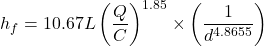Due to limiting effects from the friction coefficient (C) the Hazen-Williams approach is simply an approximation of losses. The coefficient does not account for viscosity changes and is valid for fluids with a kinematic viscosity of around 1.13 centistokes. As a result, it is accurate for only water around room temperature. In addition, it does not consider the effects of Reynolds number and is valid for flow speeds less than 10 feet/s and pipe diameters greater than 2 inches.

Nevertheless, the use of this equation is still common in sprinkler systems and water supply networks. When combined with the appropriate friction coefficient for the pipe material, satisfactory results may be achieved. Beyond these applications, the Darcy-Weisbach equation is preferable to the Hazen-Williams equation.

## Darcy-Weisbach Equation

The Darcy-Weisbach equation uses a more accurate friction coefficient vs Hazen-Williams as it comes from the Moody diagram. This diagram incorporates Reynold’s numbers, thus offering a more robust equation across wider range of viscosity, flow speed, and pipe diameter sizes. Darcy-Weisbach predicts head loss (Δhf) as a function of the pipe length (L), mean flow velocity (u), friction factor (f), gravity acceleration (g), and flow diameter (D).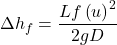This equation provides accurate results for steady state and incompressible flow. Also, the Darcy-Weisbach friction factor depends on the Reynolds number and the pipe roughness, as specified from the Moody diagram. Some Moody charts specify an alternative, which is the Fanning friction factor (Cf).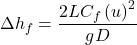Both friction factors carry identical information, but the Fanning factor is a quarter of the Darcy-Weisbach factor. So, it is necessary to find out which one a Moody chart specifies prior to use.

## The Use of Hazen-Williams vs Darcy-Weisbach

The Darcy-Weisbach equation is an improvement on the Hazen-Williams with several differences as the table below shows.

## Hazen-Williams vs Darcy-Weisbach vs Others

Apart from Hazen-Williams and Darcy-Weisbach equations, there are others that serve in estimating head/pressure loss in flow systems. The following sections highlight their differences from these popular approaches.

### Hazen-Williams vs Manning

Manning equation provides a versatile formula that is popular in computing several parameters about water resources. Unlike Hazen-Williams vs Darcy-Weisbach, the Manning equation computes flow in open channels. In addition, it can derive the capacity of a pipe, check the performance of an area-velocity flow meter, and compute friction losses. The basic form of the Manning equation relates the flow rate (Q), Manning’s roughness coefficient (n), hydraulic radius (Rh), channel slope (S), and a unit conversion factor (k).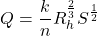For S.I. units, the unit conversion factor has a value of 1, but when using imperial units the value is 1.49. Moreover, the hydraulic radius is a ratio of the flow cross-sectional area (A) to the wetted perimeter of the channel (P). This variable accounts for the channel geometry and gives the equation the flexibility of dealing with diverse geometries for the flow channel. Computing the head loss from Manning is simply a product of the channel slope (S) and the channel length (L).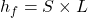Although both the Manning and Hazen-Williams equations are for calculating the flow of water, there are fundamental differences, which the following table highlights.

### Hazen-Williams vs Colebrook-White

The Colebrook-White equation is ideal for calculating the Darcy-Weisbach friction coefficient in turbulent flows that exceed Re = 4,000. It expresses this friction factor (f), as a function of the hydraulic diameter (Dh), Reynold’s number (Re), and absolute pipe roughness (e).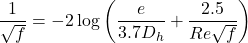Because the friction factor is on both sides of the equation it requires iterative computation. Before the computer era, achieving this was difficult. But now, this equation enjoys adoption for a variety of fluids. The table below highlights some of its differences in relation to the Hazen-Williams equation.

### Darcy-Weisbach vs Manning

The Darcy-Weisbach and Manning equations are both useful in estimating head loss. They have differences, which the following table highlights.

### Darcy-Weisbach vs Colebrook-White

Both Darcy-Weisbach and Colebrook White are useful for flow calculations in a variety of fluids. Also, they utilize the same friction factor. However, there are differences between them, which the table below outlines.